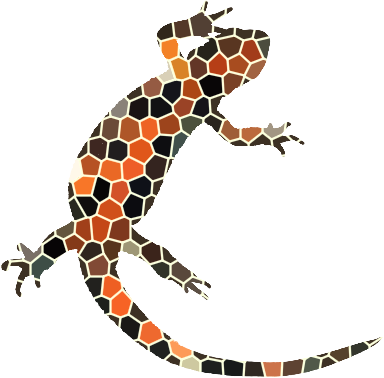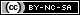Last modified: Thursday, 21-Nov-2019 02:34:57 UTC. Maintained by: Elisa E. Beshero-Bondar (eeb4 at psu.edu). Powered by firebellies.

# SVG Exercise 2

adapted with small revisions from Obdurodon.

### The text

With this assignment, you will gain experience with writing XSLT to generate SVG. We will work with an XML document from a Pitt student project on Lewis Carroll’s Alice in Wonderland, and you can download the XML file from here by right-clicking `on this`. (Don’t just click to open it in a browser and copy, which can add some browser rendering characters that will mess up your code; right click and download.)Let’s analyze the Alice XML file. The root element, `<alice>`, contains `<cast>` and `<titlePage>` child elements, both of which you can ignore, followed by twelve child `<chapter>` elements. The chapters are numbered with a `@which` attribute, e.g., ```<chapter which="1">```. Chapters contain paragraph elements (`<p>`), which contain various child elements and descendants. You are interested in the `<q>` elements inside the chapters (at various depths), which are used to tag quotes by characters in the story.

Your goal is to create a graph that charts the number of quotes by Alice herself in each chapter. You do not have to graph any character except Alice. Your X axis marks the chapters and your Y values reflect the number of `<q>` elements in each chapter that have an `@sp` attribute equal to alice. One way to graph this (as a line graph) might look something like http://newtfire.org/dh/alice_svg_output.svg. We decided to try a line graph here to indicate variation across a connected series (over time) from chapter to chapter. However, a bar graph could also make sense, too, and either approach is fine for this assignment. (When you think about SVG for your projects, think about what kinds of plots make sense: For some kinds of data that aren't connected to each other, we might want side-by-side bars to compare.)

### The XSLT Stylesheet Template for Outputting SVG

To output SVG from XML, we need to make some modifications to the default xs:stylesheet and xsl:output statements, so that the XSLT properly outputs valid W3c code that we can read in a web browser. Below is the code you need at the top of your XSLT file for this assignment. The input XML is not in the TEI namesepace, so we use the defalut XML schema, but we need to indicate the output SVG namespace (in the highlighted purple line below. The xsl:output method is set to "xml" as a default, classifying SVG as a kind of XML.

```           <?xml version="1.0" encoding="UTF-8"?>
<xsl:stylesheet xmlns:xsl="http://www.w3.org/1999/XSL/Transform" version="2.0"
xmlns:xs="http://www.w3.org/2001/XMLSchema"
exclude-result-prefixes="xs"
xmlns="http://www.w3.org/2000/svg">

<xsl:output method="xml" indent="yes"/>

```

### Working with variables

Our solution uses global variables (variables that are defined once for the entire stylesheet) as well as variables that have different values for each chapter in the book (that is, for each dot in the graph). We worked with variables already in the XSLT Exercises 5 and 6, but for a review, see https://www.w3schools.com/xml/ref_xsl_el_variable.asp or look them up in the Michael Kay book. What is new here is that we are going to use multiple variables, and most of these will help us to generate the number values we need to plot our SVG graph.

As an example of a global variable, the amount of space between chapters on the X axis (that is, the amount of horizontal space between dots) is constant for the entire SVG graph. That is, each dot is the same distance from its neighbors as other dots are from their neighbors. If we want that distance to be, say, 100 pixels, we can define a global variable with something like:

`<xsl:variable name="Xinterval" select="100"/>`

We can then refer to the `\$Xinterval` variable when we need to space out our dots while plotting them. This is a convenience variable, which means that it wasn’t absolutely necessary, since we could instead have written 100 wherever we need it. What’s convenient about the variable is that if we later decide to change the value, it could be hard to find a number inside XSLT or SVG code. If we’ve put the number in a variable definition, we can find and change it more easily. Global variables are defined as children of the root `<xsl:stylesheet>` element. We usually write them immediately after our `<xsl:output>` element, so that we can find them easily.

We can also set convenience variable values that may be different for different chapters (different dots). These are not global variables because they don’t always have the same value. For example, in the code that draws the dots for each chapter, we can set the X position of a dot with something like:

`<xsl:variable name="Xpos" select="position() * \$Xinterval"/>`
using the position() function to return the position of each chapter, or just use the number value coded in the `@which` attribute:
`<xsl:variable name="Xpos" select="@which * \$Xinterval"/>`

This is using the symbol for multiplication (`*`). We can use the following basic numeric operators in XPath and XSLT: `+` (for adding one value to another), `-` (for subtracting one value from another), and `*` (for multiplying one value by another). For division, we cannot use the forward slash because that literally means taking an XPath step, so we use `div` (to divide one value by another), and one more: `mod` to return the remainder after dividing one value by another. (Here is a handy page to look at examples of each notation.) In our example above, if we’ve previously defined `\$Xinterval` as equal to 100, our code for multiplication will set the value of the `\$Xpos` variable to "100" for chapter 1 (the first of the twelve chapters, and therefore in position #1), to "200" for chapter 2, etc. (You will also calculate the Y position and assign that value to the variable `\$Ypos`.) We can then plot the dot with something like:

`<circle cx="{\$Xpos}" cy="{\$Ypos} r="5" fill="red">`

Note the curly braces, which create an attribute value template (AVT) that causes the variable to be interpreted and its value to be output. (If you don’t use an AVT, you’ll set your X position to a literal value of `\$Xpos`, which is invalid because the X position of an SVG `<circle>` element must be numeric.) You don’t need curly braces for the `@r` attribute (the radius of the circle) if you just plug in a literal number for it like we did here, and you don’t need it for the `@fill` (color) because that value should be a literal color name or some other representation of a color. If you want to, though, you could store either of these values in global variables and call on them here, too, using curly braces.

Putting the X position into a variable is handy because you're going to need it both to position the dot and to position the chapter label (see the labels on the X axis on our sample output at the link above). If you calculate the position for each chapter once and stash it in a variable, you reuse the variable to position two things without having to redo the calculation.

### Writing the XSLT

Writing SVG with XSLT almost always involves using the pull approach, which selects for just the bits of data you need from your source XML file. It always helps to look at an example file to get started, and we have prepared one that will help show you how we plotted an X and Y axis and worked with XPath to make some estimates for how big our plot would be: See this example starter XSLT file from the Fall 2019 course’s Undertale project. (It may help to open this in oXygen and read my comments, while you're preparing this assignment.)

We've usually presumed for this plot that you'd want to spread the chapters of Allice along the X axis, and plot the number of times Alice speaks to up up the Y axis. But you should feel free to flip this around and plot it the way we did the Undertale graph in class. (This is up to you.)

After you create the SVG superstructure, plot the X and Y axes, and add some labels to the plot, you can draw the dots, the lines between dots, and the labels on the X (or Y) axis in one of two ways:

• You can use `<xsl:apply-templates select="descendant::chapter">`. If you apply templates to chapters, you’ll need two templates: a template for the document node, in which you’ll create the SVG superstructure, and a second template that matches `<chapter>` elements.
• Or you can use `<xsl:for-each select="descendant::chapter">`. If you use the `<xsl:for-each>` strategy, you’ll need only one template in your XSLT file for the document node, and you’ll put the `<xsl:for-each>` inside that.

Whether you use `xsl:apply-templates` or `xsl:for-each` to process the chapters, you will be drawing a dot (using an SVG circle element) when you process each chapter. The `@cx` coordinate for your circles needs to advance across your X axis regularly with each chapter by its number, and the `@cy` needs to represent the count of Alice’s speeches within the chapter you are processing.

#### What do you want to make: a bar graph or a line graph?

This is totally up to you, and we will show you both ways in class. The decision you make has a lot to do with whether you consider speech events to be a continuous activity throughout a novel, so you want to plot lines that show slopes that incline or decline. But you might prefer to see each chapter plotted separately.
##### Bar Graph:

We usually find it easiest to make bar graphs using the SVG `line` element, and giving it a `@stroke-width` attribute that basically makes a thick line. (We find that dealing with the height and width attributes of an SVG rectangle element can be a little challenging, but feel free to use the SVG rectangle if you wish.) You will need to plot lines that run from the axis up (or out) to the circle you plotted.

##### Line Graph:

To draw connecting lines between the dots, you’ll need to access information about two chapters at once. One will be the one you’re processing; the other will be either the one before or the one after. There are several ways to do that, and we’ll talk about them when we go over the solution, but whatever you do, note that with twelve chapters you have twelve dots but only eleven connecting lines. This means that one chapter (either the first or the last, depending on how you structure your code) will have to be treated differently from the others. For that reason, you may find it useful to test whether you’re at the beginning or end of the sequence of chapters with `<xsl:if>`.

Once we generate the line graph plotting the number of times Alice speaks in each chapter, we might want to see how those values compare with the number of speeches by all the other characters combined, that is, speaking parts by everyone who is not Alice. Our solution uses the `not()` function to collect all the non-Alice speeches. We plotted a second line graph to superimpose on the first so we could compare the two sets of data, and chose different colors for the two lines. Our sample output plot looks like this, but you should improve on it by adding a title and a legend to finish labeling the graph. compares that of all the with all the other characters besides Alice,